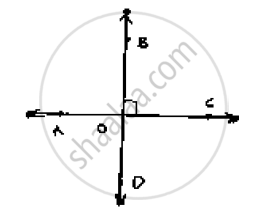# If One of the Four Angles Formed by Two Intersecting Lines is a Right Angle, Then Show that Each of the Four Angles is a Right Angle. - Mathematics

If one of the four angles formed by two intersecting lines is a right angle, then show that
each of the four angles is a right angle.

#### Solution

Given,

AB and CD are two lines intersecting at O such that

∠BOC = 90°

∠AOC = 90°, ∠AOD = 90° and ∠BOD = 90°

Proof:

Given that ∠BOC = 90°

Vertically opposite angles are equal∠BOC = ∠AOD = 90°

∠AOC, ∠BOC are Linear pair of angles

∠AOC + ∠BOC = 180°       [LinearPair]

⇒∠AOC + 90° = 180°

⇒ ∠AOC = 90°

Vertically opposite angles

∴ ∠AOC = ∠BOD = 90°

Hence, ∠AOC = ∠BOC = ∠BOD = ∠AOD = 90°

Concept: Concept of Parallel Lines
Is there an error in this question or solution?

#### APPEARS IN

RD Sharma Mathematics for Class 9
Chapter 10 Lines and Angles
Exercise 10.3 | Q 5 | Page 22